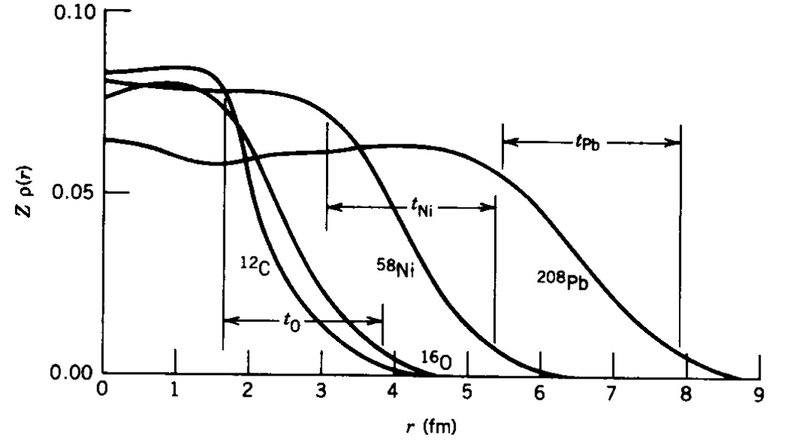# Distribution of nucleons in the nuclei

• I
What does this diagram tell us about the distribution of nucleons in the nuclei? - The diagram is from Krane Introductory Nuclear Physics

I know that nucleons don't congregate around a central part of a nucleus, but instead have a constant distribution throughout. i.e. The number of nucleons per unit of volume is fairly constant.

I'm not really sure why they all taper off the way they do though. Smaller mass number particles tend to taper off quicker, while larger mass number particles taper off slower. I'm not really sure why this is.#### Attachments

BvU
Homework Helper
I know that nucleons don't congregate around a central part of a nucleus, but instead have a constant distribution throughout. i.e. The number of nucleons per unit of volume is fairly constant
That would give horizontal lines in the diagram that drop to zero at some nucleus radius.
The densitties don't drop off sharply but taper out to zero because the nucleons are bound together by the strong force that has a very limited range (the 208Pb is the largest stable nucleus). So the nucles is a dense but but moving packing, meaning that just like with electron orbits around the nucleus, there is no sharp outer bound.

So the nucleons have a fairly constant distribution in the nucleus, but the distribution tapers off at the range of the strong force. For nuclei with larger mass number, A, this tapering off is a much longer tail?

BvU